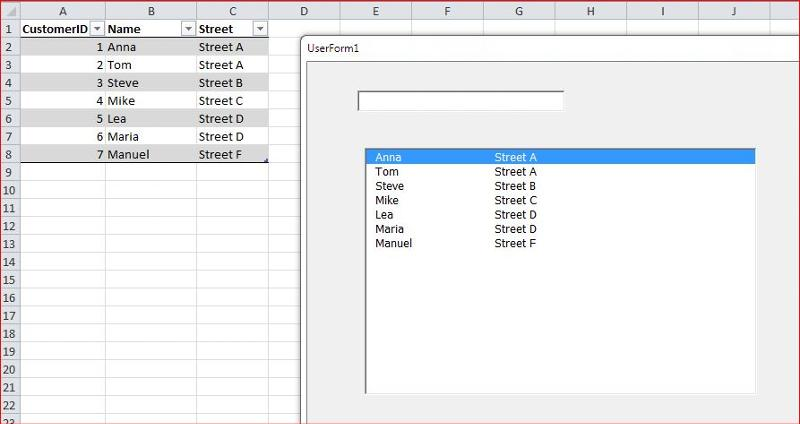# Excel VBA: Real-Time Search or Filtering in ListboxMassimo Scola used Ask the Experts™
on
I have a listbox with many records and I would like to have the ability to search inside the listbox.

The Listbox has several columns: Column 1 is the CustomerID, column 2 is the name and so on.The following code searches inside the listbox, but only in the first column - which is the ID.
How do I change the code so that it searches inside the second column?
``````Private Sub txtSearch_Change()
Dim strText As String
Dim i As Long

strText = LCase(txtSearch.Text)
With ListBox1
For i = 0 To .ListCount - 1
If LCase(Left\$(.List(i), Len(strText))) = strText Then Exit For
Next i
If i = .ListCount Then
' No matching item was found, select nothing
.ListIndex = -1
Else
' A match was found, select it
.ListIndex = i
End If
End With
End Sub
``````

Is it also possible to filter the listbox as I type?

Thanks
example.xlsm
Comment
Watch Question

Do more withEXPERT OFFICE® is a registered trademark of EXPERTS EXCHANGE®
Top Expert 2016
Commented:
Hi,

pls try

``````Private Sub txtSearch_KeyUp(ByVal KeyCode As MSForms.ReturnInteger, ByVal Shift As Integer)

Dim strText As String
Dim i As Long

strText = LCase(txtSearch.Text)
With ListBox1
For i = 0 To .ListCount - 1
If LCase(Left\$(.List(i, 1), Len(strText))) = strText Or LCase(Left\$(.List(i, 2), Len(strText))) = strText Then Exit For
Next i
If i = .ListCount Then
' No matching item was found, select nothing
.ListIndex = -1
Else
' A match was found, select it
.ListIndex = i
End If
End With
End Sub
``````
Regards
Software Engineer

Commented:
OK this works.  I have two questions

1. )Is there something like a wildcard operator which I can use if I only know part of the name?

2) If I want to filter the data, would I have to make use of arrays? Or what is the approach?
Top Expert 2016

Commented:
1) instead of Left use Instr
``````InStr(LCase(.List(i, 1)), strText)
``````

2)then try
``````Private Sub txtSearch_KeyUp(ByVal KeyCode As MSForms.ReturnInteger, ByVal Shift As Integer)

Dim rw
Dim strText As String

strText = LCase(txtSearch.Text)
Dim rng As Range
Set rng = Range("Customers")
With ListBox1
.RowSource = ""
.ColumnCount = 3
For Each rw In rng.Rows
If InStr(LCase(Cells(rw.Row, 2)), strText) Or InStr(LCase(Cells(rw.Row, 3)), strText) Then